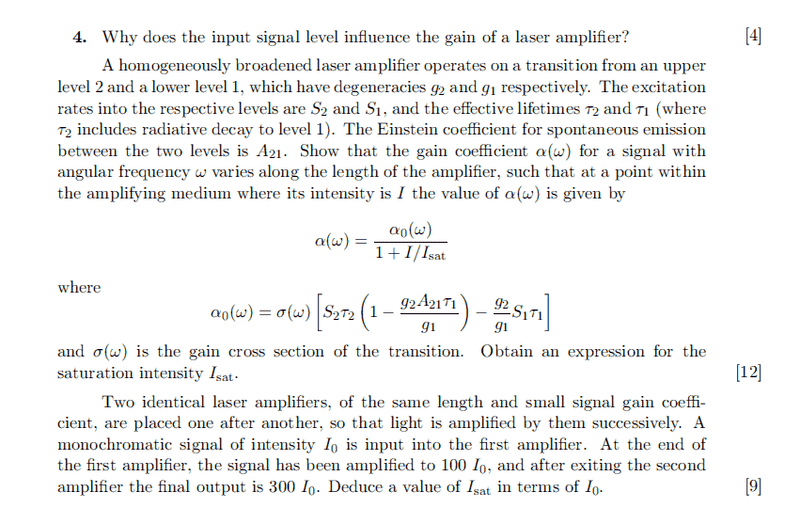# Laser saturation and gain

## Homework Statement

[/B]
(a) Why does input intensity affect gain?
(b) Derive the expression
(c) Find saturation intensity in terms of input intensity## The Attempt at a Solution

Part(a)
Consider a narrow band radiation as an input, where its bandwidth is much smaller than the spectral with of transitions, so in general
$$\frac{dN_2}{dt} = S_2 - (N_2B_{21}-N_1B_{12}) \int g_H(\omega - \omega_0) \rho(\omega) d\omega + \cdots$$
$$= S_2 - N^{*} \int B_{21} g_H(\omega - \omega_0)\rho(\omega) d\omega + \cdots$$
$$= S_2 - N^{*}\sigma_{21}(\omega_L - \omega_0) \frac{I}{\hbar \omega_L}$$

Thus, we see that rates depend on input intensity ##I_T##, which influences the gain on a laser amplifier when we solve for the steady state solutions.

Part(b)
Bookwork. Managed to derive it.
$$\alpha(\omega) = \frac{\alpha_0(\omega)}{1 + \frac{I}{I_{sat}}}$$
where saturation intensity is ## I_{sat} = \frac{\hbar \omega_L}{\sigma_{21} \tau_R}## and relaxation time is ##\tau_R = \tau_2 + \frac{g_2}{g_1} \tau_1 (1- \tau_2A_{21})##.

Part(c)
The equation for intensity is given by
$$\frac{dI}{dz} = \alpha I = \frac{\alpha_0}{1 + \frac{I}{I_{sat}}}I$$
which may be integrated to give
$$ln \left( \frac{I(z)}{I_{(0)}} \right) + \frac{I_{(z)} - I_{(0)}}{I_{sat}} = \alpha_0 z$$

At low intensity where ##I_{(z)} << I_{sat}##, the equation becomes ##I_{(z)} = I_{(0)} = e^{\alpha_0 z}##.
At high intensity where ##I_{(z)} \approx I_{(0)}##, the equation becomes ##I_{(z)} = I_{(0)} + \alpha_0 I_{sat} z##.

Thus initially the beam intensity is weak, then it becomes strong, so
$$e^{\alpha_0 z} = 100$$
$$200I_0 = \alpha_0 I_{sat}z$$

## Answers and Replies

bumpp

would appreciate any input on the last bit, many thanks in advance!

bumpp

bumpp on last part

bumpp

bumpp

blue_leaf77
Science Advisor
Homework Helper
For part (c) build two different equations, each of which relates the output intensity, input intensity, and distance (hint: you have derived this equation). Then to each one of them, input the different conditions, one is for after travelling the first amplifier and the other after travelling both amplifiers. It's then straightforward to derive the expression for the saturation intensity in term of only input intensity.

•unscientific
For part (c) build two different equations, each of which relates the output intensity, input intensity, and distance (hint: you have derived this equation). Then to each one of them, input the different conditions, one is for after travelling the first amplifier and the other after travelling both amplifieTrs. It's then straightforward to derive the expression for the saturation intensity in term of only input intensity.

You absolutely right. I have been making unnecessary approximations. Solved this question.